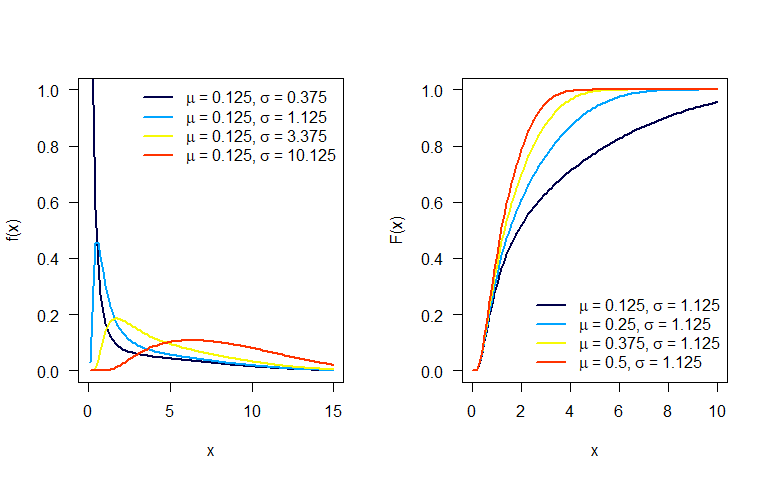# FWE distribution

#### 2022-12-21

library(RelDists)
#>
#> DEoptim package
#> Differential Evolution algorithm in R
#> Authors: D. Ardia, K. Mullen, B. Peterson and J. Ulrich
#>
#> Attaching package: 'BBmisc'
#> The following object is masked from 'package:base':
#>
#>     isFALSE
#> Package 'GA' version 3.2.2
#> Type 'citation("GA")' for citing this R package in publications.
#>
#> Attaching package: 'GA'
#> The following object is masked from 'package:utils':
#>
#>     de
#>
#>
#> ><<<<<<<<<<<<<<<<<<<<<<<<   EstimationTools Version 4.0.0   >>>>>>>>>>>>>>>>>>>>>>>><
#>   Feel free to report bugs in https://github.com/Jaimemosg/EstimationTools/issues

# Flexible Weibull extension distribution

This distribution was proposed by Bebbington (2007). The probability density function $$f(x)$$ and cumulative density function $$F(x)$$ are given by:

$f(x) = \left( \mu+ \frac{\sigma}{x^2} \right) e^{\mu x - \sigma / x} \exp \left( -e^{\mu x - \sigma / x} \right),$

and

$F(x) = 1 - \exp[-e^{\mu x - \sigma / x}], \quad x > 0.$

respectively, where $$\mu > 0$$, $$\sigma > 0$$ and $$x > 0$$.

Next figure shows possible shapes of the $$f(x)$$ and $$F(x)$$ for several values of the parameters.Bebbington, M., C. D. Lai, and R. Zitikis. 2007. “A Flexible Weibull Extension.” Reliability Engineering & System Safety 92 (6): 719–26.